# Week 12: Proving NP-Completeness¶

In :
from tock import *
settings.display_direction_as = "alpha"
from cooklevin import *


## Tuesday¶

Last time, we showed that the language $\mathit{TIME}_{\mathsf{NTM}}$ was NP-complete:

$$\mathit{TIME}_{\mathsf{NTM}} = \{ \langle N, w, \texttt{1}^t\rangle \mid \text{NTM N accepts w within t steps} \}.$$

Today, we start to consider other NP-complete languages.

### Proving NP-completeness using reductions¶

Now, we take the next step towards more real-world problems by showing that a simpler problem called 3SAT is NP-complete.

If we wanted to prove that 3SAT is NP-hard "from scratch", we'd have to give a polynomial-time reduction from any NP language $A$ to 3SAT: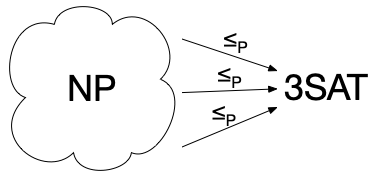But now that we've proven that $\mathit{TIME}_{\mathsf{NTM}}$ is NP-complete, our task becomes slightly easier. We no longer have to show a reduction from every NP language $A$ to 3SAT; we know that every NP language reduces to $\mathit{TIME}_{\mathsf{NTM}}$, so it's enough to show a reduction from $\mathit{TIME}_{\mathsf{NTM}}$ to 3SAT, and we automatically get a reduction from every NP language $A$ to 3SAT: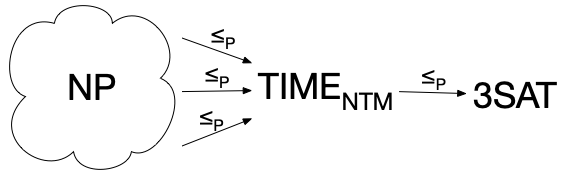This is possible because polynomial-time reducibility is transitive. Here's a pictorial proof of this fact: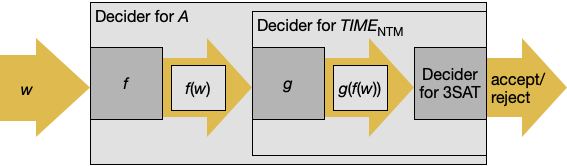Once we've proven 3SAT NP-complete, we can prove that (say) CLIQUE is NP-complete by giving a reduction from 3SAT to CLIQUE, and so on.

Here's a picture of the reductions proven in the book (with the addition of $\mathit{TIME}_{\mathsf{NTM}}$):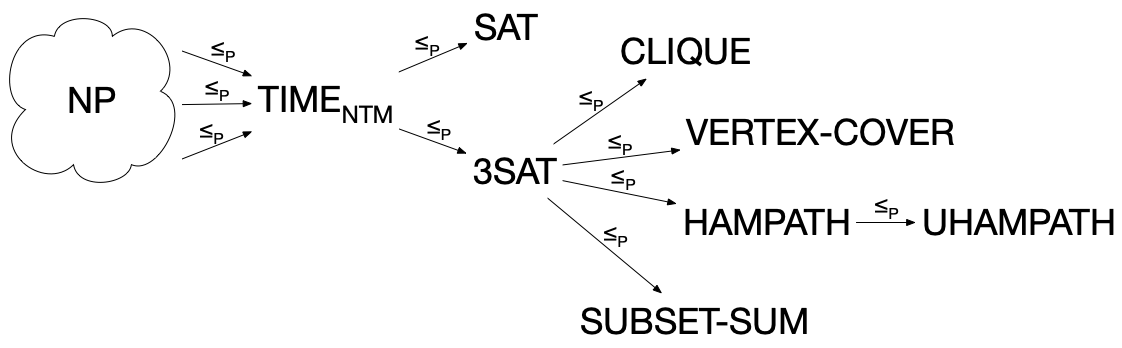So we can revise our skeleton NP-completeness proof. To prove that a language $B$ is NP-complete by reduction from another NP-complete language $A$, you must do the following:

• To prove that $B$ is in NP, you either:
• Give a polynomial-time deterministic verifier for it, or
• Give a polynomial-time NTM for it.
• To prove that $B$ is NP-hard, assume that $B$ can be decided in polynomial time by $R$, and construct a polynomial-time decider for $A$: $S={}$"On input $w$, 1. Compute $f(w)$. 2. Run $R$ on $f(w)$. 3. If $R$ accepts, accept; else, reject." You fill in:
• Define a mapping $f$ from instances of $A$ to instances of $B$.
• Prove that $w \in A$ iff $f(w) \in B$.
• Prove that $f$ runs in polynomial time.

### The Cook-Levin Theorem¶

Read Section 7.4, "The Cook-Levin Theorem."

A Boolean formula is made out of variables, negation ($\neg x$ or $\bar x$), conjunction ($\land$), and disjunction ($\lor$). A formula $\phi$ is satisfiable iff there is a way to assign true or false values to variables such that $\phi$ is true.

The Boolean satisfiability problem (SAT) is

$$\{ \phi \mid \text{\phi is satisfiable} \}.$$

Now we want to show that SAT is NP-complete.

#### The big picture¶

This is a really long proof. I think it's helpful to first look at how it lines up with our skeleton proof.

First, we have to show that SAT is in NP, which is easy. Let's get that out of the way: it's enough to say that we can check a truth-assignment for $\phi$ in time linear in the length of $\phi$.

To show that SAT is NP-hard, we need to show that if SAT was decidable in polynomial time by a TM $R$, we would be able to decide $\mathit{TIME}_{\mathsf{NTM}}$ in polynomial time, in three steps: On input $\langle N, w, \texttt{1}^t\rangle$,

1. Convert $\langle N, w, \texttt{1}^t\rangle$ to a Boolean formula $\phi$.
2. Run $R$ on $\phi$.
3. If $R$ accepts, accept; else, reject.

So all the work is in defining a function $f$ that converts $\langle N, w, \texttt{1}^t\rangle$ to a formula $\phi$, showing that that $\phi$ is satisfiable iff $N$ accepts $w$ within $t$ steps, and showing that $f$ runs in polynomial time.

#### The medium picture¶

Given $N$ and $w$, can can construct a tableau, which is a two-dimensional representation of a computation history for $N$ on $w$. The first row is the start configuration, and the following rows are the steps of the run. Each row is delimited by # on the left and right. Finally, if a row is an accepting configuration, the same configuration is repeated in the rows below it (this detail is missing from the book, but something like it seems necessary).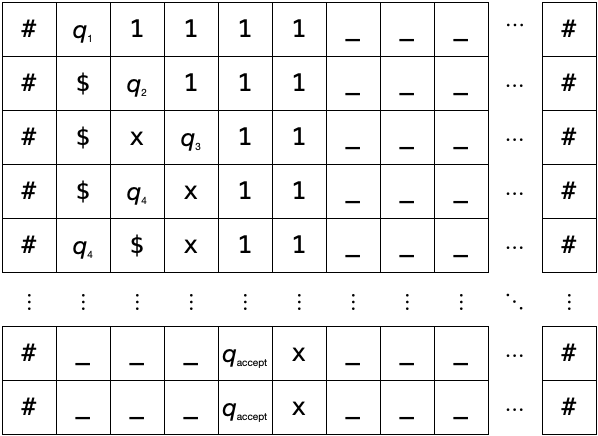Because we only need to simulate $N$ up to $t$ steps, we can fix the size of the tableau in terms of $t$. There are $t+1$ rows: one row for the start configuration, plus one row for each step. And there are $t+4$ columns, because in $t$ steps, the head could move as far right as the $(t+1)th$ cell, plus one for the state, plus two for the #'s.

Now we need to write a giant formula $\phi$ that checks whether the tableau is an accepting computation history for $N$ on $w$. The variables of this formula are $x_{i,j,s}$, where $i$ is a row number, $j$ is a column number, and $s$ is either a tape symbol or a state. If $x_{i,j,s}$ is true, that means that cell $i,j$ contains symbol $s$. It's $\phi$'s job to check that a truth-assignment encodes a well-formed, accepting tableau.

The formula $\phi$ has four parts: $\phi = \phi_{\mathrm{cell}} \land \phi_{\mathrm{start}} \land \phi_{\mathrm{move}} \land \phi_{\mathrm{accept}}$. The purpose of these four parts is:

• $\phi_{\mathrm{cell}}$: Each cell contains exactly one symbol.
• $\phi_{\mathrm{start}}$: The first row of the tableau is the initial configuration of $N$.
• $\phi_{\mathrm{move}}$: Each row (except the first) is a configuration that legally follows the row above it according to $N$'s rules.
• $\phi_{\mathrm{accept}}$: The last row is an accepting configuration.

#### Every cell has exactly one symbol¶

Let $C = Q \cup \Gamma \cup \{\#\}$, the set of all possible symbols in a tableau cell. We ensure that every cell has exactly one symbol with the subformula:

\begin{align*} \phi_{\text{cell}} = \bigwedge_{1 \leq i \leq t+1} \bigwedge_{1 \leq j \leq t+4} &\bigg[ && \text{for each cell,} \\ & \bigvee_{s \in C} x_{i,j,s} \land {} && \text{each cell has at least one symbol} \\ & \bigwedge_{s,s' \in C, s \neq s'} \neg (x_{i,j,s} \land x_{i,j,s'}) && \text{and no cell has two symbols} \\ &\bigg] \end{align*}

#### The first row is a start configuration¶

\begin{align*} \phi_{\text{start}} = & x_{1,1,\#} \land {} && \text{left boundary} \\ & x_{1,2,q_0} \land{} && \text{start state} \\ & \bigwedge_{1 \leq i \leq n} x_{1,i+2,w_i} \land{} && \text{input string} \\ & \bigwedge_{n+1 \leq i \leq t+1} x_{1,i+2,\_} \land{} && \text{blank symbols} \\ & x_{1,t+4,\#} && \text{right boundary} \end{align*}

#### Each row (except the first) follows from the previous row¶

This is the most complicated part of the formula.

\begin{align*} \phi_{\text{move}} = \bigwedge_{1 \leq i \leq t} \bigwedge_{2 \leq j \leq t+1} &\bigg[ && \text{for each $2\times3$ window} \\ & \bigvee_{\text{$a_1, \ldots, a_6$ is a legal window}} x_{i,j-1,a_1} \land x_{i,j,a_2} \land x_{i,j+1,a_3} \land x_{i+1,j-1,a_4} \land x_{i+1,j,a_5} \land x_{i+1,j+1,a_6} && \text{the window is legal} \\ &\bigg] \end{align*}

The book just gives a few examples of legal windows (Figure 7.39) but doesn't list them all out. If you like details to be completely worked out, here they are.

This is a legal window:

abc
abc

where a, b, and c are tape symbols, #, or $q_{\text{accept}}$.

Recall that if there is a transition $q \xrightarrow{b \rightarrow c, \text{L}} r$, then the configuration $uaqbv$ yields configuration $uracv$, where $u$ and $v$ are strings of tape symbols. So the following are all legal windows:

xya  yaq  aqb  qbx  bxy
xyr  yra  rac  acx  cxy

where $x$ and $y$ are any tape symbols or #.

A special case is when the head is at the left end of the tape and tries to move left ($qbv$ becomes $rcv$).

#qb  qbx
#rc  rcx

Similarly, if there is a transition $q \xrightarrow{b \rightarrow c, \text{R}} r$, then the configuration $uaqbv$ yields configuration $uacrv$. So the following are all legal windows:

yaq  aqb  qbx  bxy
yac  acr  crx  rxy

where $x$ and $y$ are any tape symbols or #.

These 12 cases cover all possibilities -- nothing else is a legal window.

#### The last row is an accepting configuration¶

$$\phi_{\text{accept}} = \bigvee_{2 \leq j \leq t+2} x_{t+1,j,q_{\text{accept}}}$$

#### Demo¶

Here's an NTM for $\{\texttt{1}^n \mid \text{$n$is composite}\}$:

In :
m = read_csv("primes.csv")
m

In :
run(m, ["1"]*4)

In :
run(m, ["1"]*4).shortest_path()

Out:
 q1  1 1 1 q2 $ 1 1 q3$ x  1 q4 $[x] 1 1 q4 [$] x 1 1 q5s _ [x] 1 1 q5s _ x  1 q6 _ [x] $1 q6 [_] x$ 1 q7 _ [x] $1 q5x _ _ [$] 1 q5x _ _ $ q6 _ _ [$] x q6 _ [_] $x q7 _ _ [$] x q5s _ _ _ [x] q5s _ _ _ x [_] accept _ _ _ [x] _

The running time of this NTM actually isn't bad -- bounded above by $3n^{3/2}$:

In :
# Print n, actual running time, upper bound on running time
for n in range(20):
r = run(m, ["1"] * n)
if r.has_path():
print(n, len(r.shortest_path().configs), 3*n**1.5)

4 18 24.0
6 28 44.090815370097204
8 38 67.88225099390857
9 53 81.0
10 48 94.86832980505137
12 58 124.70765814495917
14 68 157.14961024450554
15 95 174.28425057933376
16 78 192.0
18 88 229.10259710444143


Now we construct the formula corresponding to the run of this machine on 0000:

In :
phi = make_phi(m, ["1"]*4, 24)
print(phi.size()) # number of variables and operators in phi

9116869


It's too big to print, but we can look at the beginning:

In :
print(str(phi)[:1000], '...')